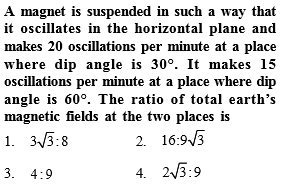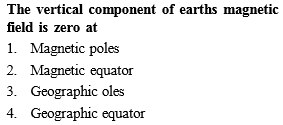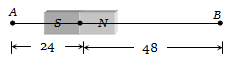You’ve reached the end of your free Videos limit.
#10 | Earth's Magnetism
(Physics) > Magnetism and Matter
Related Practice Questions :Complete Question Bank + Test Series
Complete Question Bank

Difficulty Level:Complete Question Bank + Test Series
Complete Question Bank

Difficulty Level:

A bar magnet of length 3 cm has points A and B along its axis at distances of 24 cm and 48 cm on the opposite sides. Ratio of magnetic fields at these points will be(a) 8                               (b)$\frac{1}{2\sqrt{2}}$

(c) 3                               (d) 4

Complete Question Bank + Test Series
Complete Question Bank

Difficulty Level:

If the angles of dip at two places are 30o and 45o respectively, then the ratio of horizontal  components of earth's magnetic field at the two places will be

(a) $\sqrt{3}:\sqrt{2}$                       (b) $1:\sqrt{2}$

(c) $1:\sqrt{3}$                          (d) 1:2

Complete Question Bank + Test Series
Complete Question Bank

Difficulty Level:

A line passing through places having zero value of magnetic dip is called

(a) Isoclinic line             (b) Agonic line

(c) Isogonic line             (d) Aclinic line